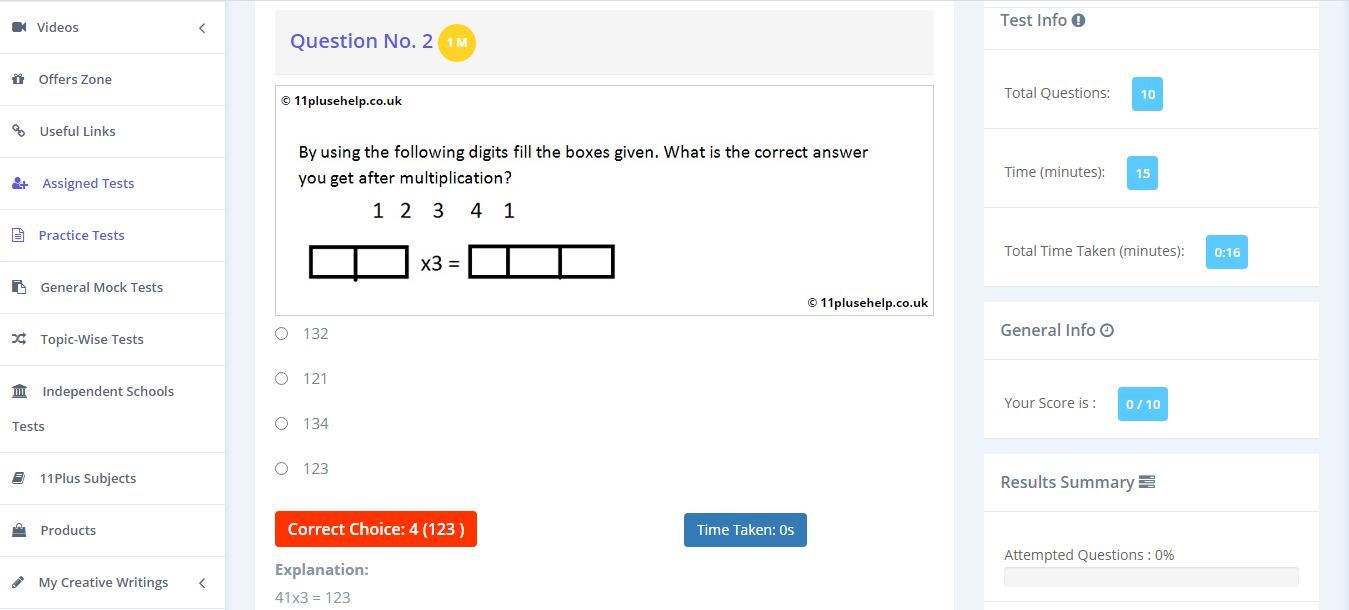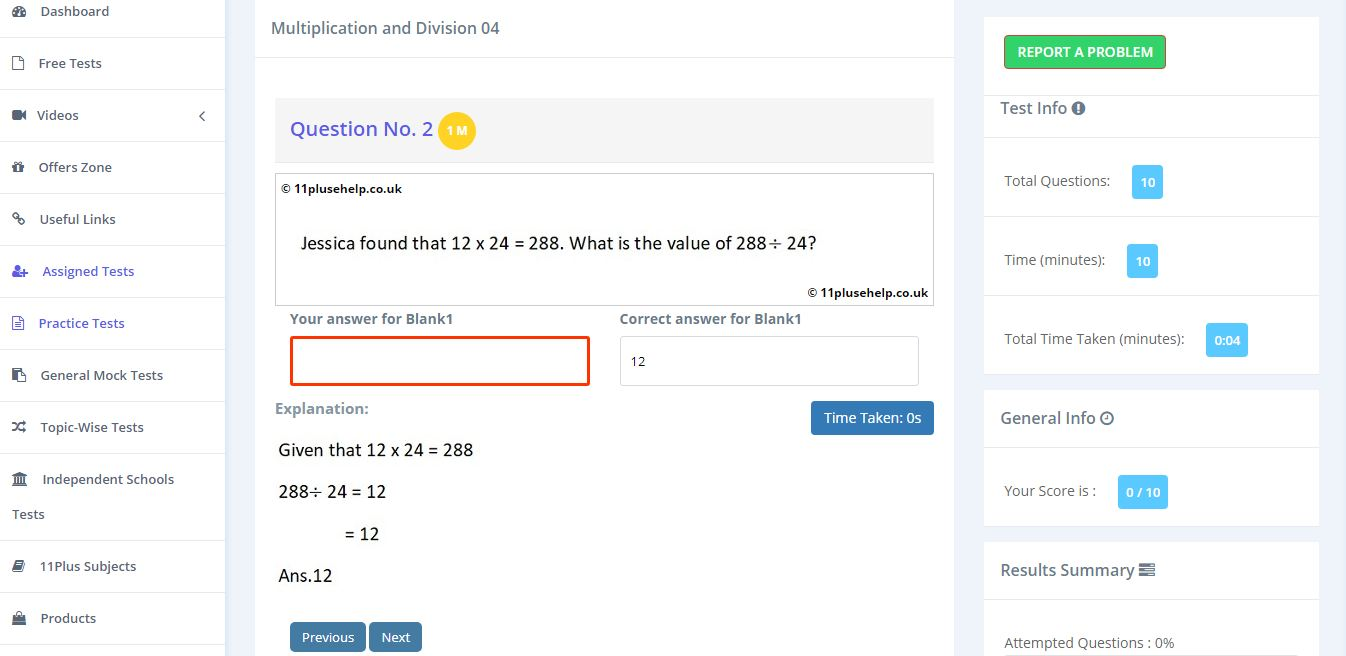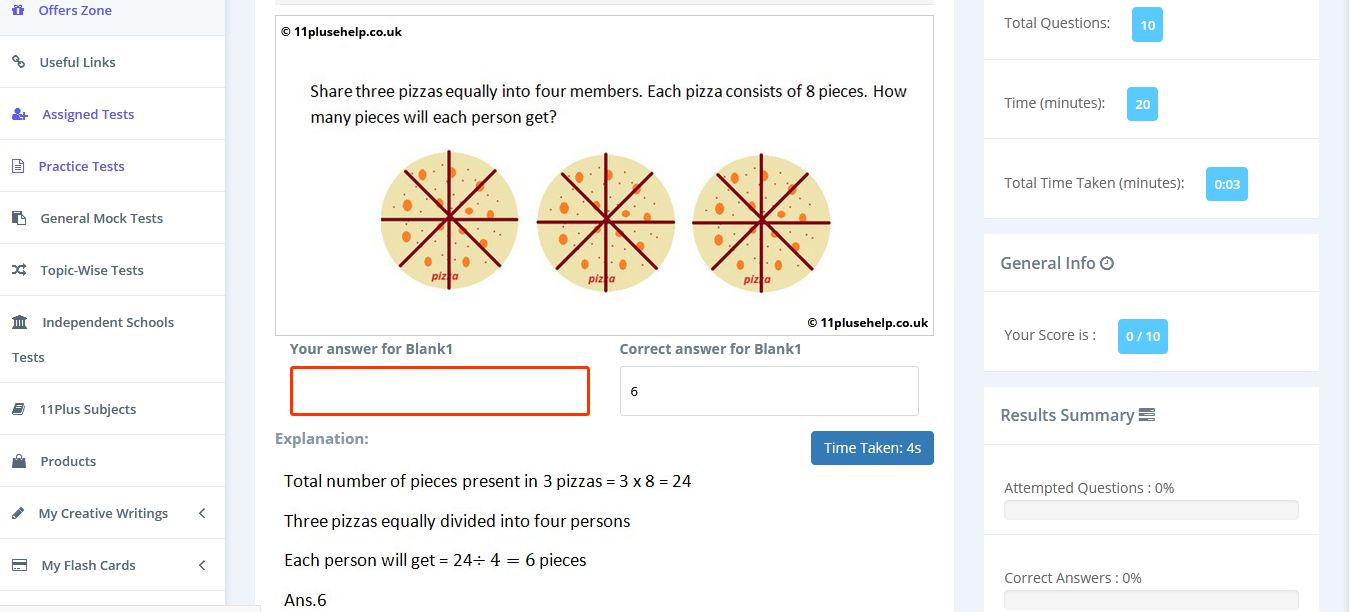Latest Results:

11 Plus Addition Subtraction Multiplication and Division

Generally, four arithmetical operations are applied to numbers: addition, subtraction, multiplication, and division. They even have their own signs like +, -, x and ÷ for addition, subtraction, multiplication and division, respectively.

Addition or sum operations are performed to add some quantity to another quantity. It is considered an increment operator. It uses the symbol + (Plus).

Let’s see an example of addition.

Example1: What is the value of £ 100 + £ 50?

Sol: It is asked to find the value of £ 100 + £ 50 = £ 150.

Note: While adding a term known as carry comes into the picture. Suppose you want to add 7 to 18, then carry will be generated, this is because 7 + 8 is more than 9, it is 15. So one is added to the 10s place to make it 25.

Subtraction:

Subtraction or difference operations are performed to remove some quantity from another quantity. It is considered as a Decrement operator. It uses the symbol (minus).

Let’s see an example of subtraction.

Example1: What is the value of £ 100 - £ 50?

Sol: It is asked to find the value of £ 100 - £ 50 = £ 50.

Note: While Subtracting, a term known as borrow, comes into the picture. Suppose you want to perform 27 - 8, then borrow will be required, this is because 8 is greater than 7. So 1 from 10s place is taken to make units place 17.

Multiplication:

Multiplication or product operations are performed to multiply some quantity with another quantity. It uses the symbol x (into), sometimes *is also used.

Let’s see an example for Multiplication.

Example1:

What is the value of 10 x 5?

Sol: It is asked to find the value of 10 x 5 = 50. Which can also be found as 10 + 10 + 10 + 10 +10 = 50, instead of writing many times we use this operator.

Division:

Division operations are performed to divide some quantity into the required number of quantities. It uses the symbol ÷ (by), sometimes / is also used. It is used in the concept of sharing.

Let’s see an example for Division.

Example1: What is the value of 10 ÷ 5?

Sol: It is asked to find the value of 10 ÷ 5 = 2. It means ten items are divided into five sets, and each set will have two items.

Now consider the above example from another point of view. Let A have 10 cherries, and he has 5 sons and wants to distribute among them then 10 ÷ 5 = 2 is performed, and each son gets 2 cherries.

Some other terms are involved in the division. Let’s have a short glance at them.

Dividend ÷ Divisor = Quotient

Sometimes we cannot divide the items equally. In the above example, if 11 cherries are considered instead of 10, then after distribution, one would have leftover, and it is called Remainder.

Important facts of Arithmetic Operations :

• The quantities involved in addition and subtraction must have similar units. It means we cannot add or subtract 10km and 10kg as both the quantities are different.
• Anything added or subtracted with 0 gives same value (10 + 0 = 10; 20 – 0 = 20).
• Product of 2 minus signs results is plus (i.e. – x - = +).
• Anything multiplied with 0 gives 0 (1 million x 0 = 0).
• If dividend is 0 then quotient will be 0 (0/10 = 0).
• If dividend and divisor are equal, then the quotient will always be 1 (10/10 = 1).
• If the divisor is 0, then that division is treated as undefined (10/0 = undefined).

Some examples from our 11 Plus Maths Practice Papers:

Example:1Example:2Example:3You can access maths papers on different topics here:

https://www.11plusehelp.co.uk/11-plus-maths-practice-tests

11PluseHelp.co.uk is a one-stop shop for 11 Plus exam preparation, including 11 Plus Independent schools preparation.

You can get the complete 11 Plus Revision Pack here:

https://www.11plusehelp.co.uk/11-plus-practice-papers/11-plus-english-practice-test-papers/revision-pack

We focus on fundamentals, logic, basics and cover most of the syllabus for all 11 Plus exam patterns in the UK. For example, We cover Maths topic wise questions and fundamentals and how to apply them. We have constantly been adding practice papers regularly.

We have loads of questions useful for preparing for CEM, CSSE and GL assessment and any other 11 Plus entrance exams in the UK. Children will love to take these tests, and learning is fun at 11plusehelp.co.uk.

To access Virtual Mock Exams, please visit:

https://11plus-mockexams.co.uk/

You can access 11 Plus FREE Papers by visiting the below link:
https://www.11plusehelp.co.uk/11-plus-free-online-papers

You can access 11 Plus FREE Sample Papers by visiting the below link:
https://www.11plusehelp.co.uk/11-plus-sample-papers

11 Plus complete solution features:

https://11plusehelp.co.uk/blog/2017/05/01/11-plus-complete-solution/

Practice and Perseverance Over Genius and Talent

Thanks,

11Plusehelp.co.uk